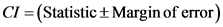# The margin of error is the error from using volunteer sampling methods.

TRUE OR FALSE The margin of error of a confidence interval is the error from
using volunteer sampling methods. True or False

General guidance

Concepts and reason
Confidence interval:
A range of values such that the population parameter can expected to contain for the given confidence level is termed as the confidence interval. In other words, it can be defined as an interval estimate of the population parameter which is calculated for the given data based on a point estimate and for the given confidence level.
Moreover, the confidence level indicates the possibility that the confidence interval can contain the population parameter. Usually, the confidence level is denoted by a. The value is chosen by the researcher. Some of the most common confidence levels are 90%, 95%, and 99%.
Margin of Error:
The margin of error is defined as a statistic which gives the amount of sampling error in the given study. Also, the margin of error tells the percentage of points that the obtained results would differ from that of the given population value.

Fundamentals

The confidence interval formula is,

Step-by-step

Step 1 of 2

The objective of the problem is obtained below.
The margin of error is obtained by multiplying the standard error with critical value. It is used to obtain the confidence interval. The sampling error can be obtained while collecting a sample.

The objective of the problem is to obtain the statement ‘margin of error of a confidence interval is the error from using volunteer sampling methods’ True or False.

Use concept of ‘Margin of Error’ to identify the statement ‘margin of error of a confidence interval is the error from using volunteer sampling methods’ True or False.

Step 2 of 2

The statement ‘margin of error of a confidence interval is the error from using volunteer sampling methods’ True or False is obtained below.
The margin of error of a confidence interval is not an error from using volunteer sampling methods. The margin of error is used to calculate the confidence interval of a parameter. The margin of error is obtained by multiplying the critical value with standard error of the statistic value.

False, the margin of error of a confidence interval is the error from using volunteer sampling methods.

The margin of error is used to estimate the confidence interval of a parameter at a required confidence level. The margin of error is product of standard error and critical value.